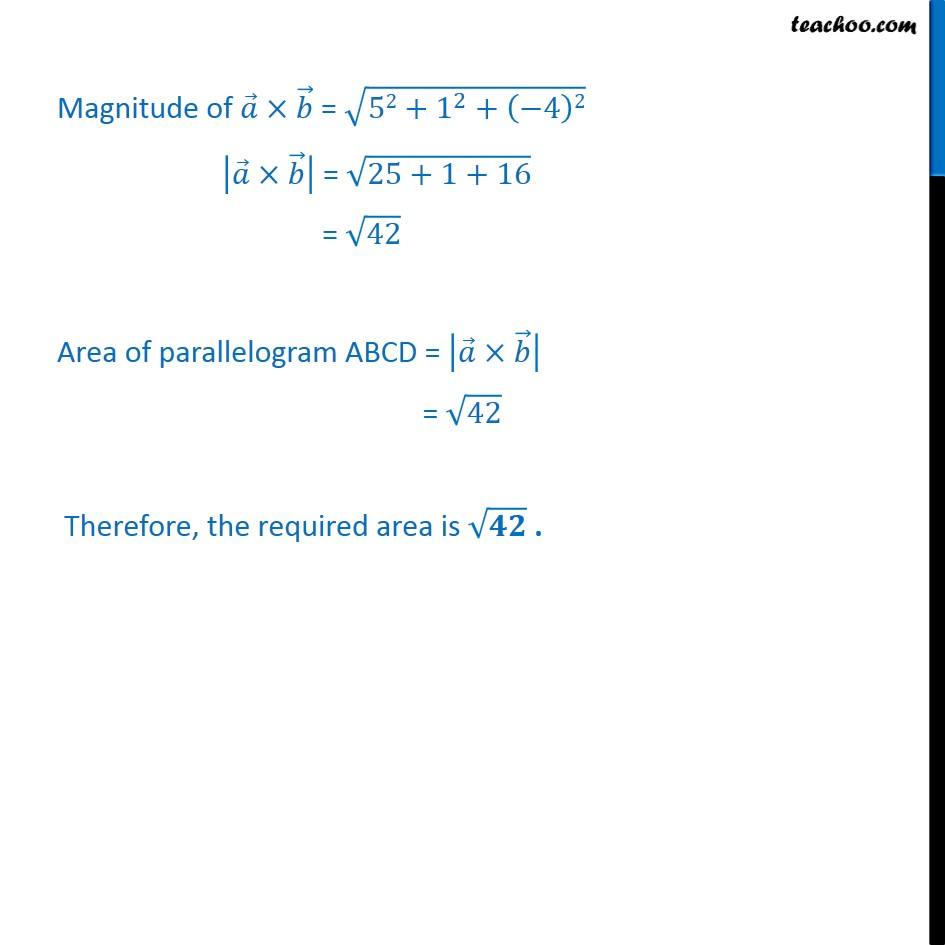1. Class 12
2. Important Question for exams Class 12
3. Chapter 10 Class 12 Vector Algebra

Transcript

Example 25 Find the area of a parallelogram whose adjacent sides are given by the vectors a = 3 + + 4 and b = + k a = 3 + 1 + 4 b = 1 1 + 1 k Area of parallelogram ABCD = = 3 1 4 1 1 1 = (1 1 ( 1) 4) (3 1 1 4) + (3 1 1 1) = (1 (-4)) j (3 4) + ( 3 1) = (1 + 4) j ( 1) + ( 4) = 5 + 4 Magnitude of = 52+ 1 2 + 4 2 = 25+1+16 = 42 Area of parallelogram ABCD = = 42 Therefore, the required area is .

Chapter 10 Class 12 Vector Algebra

Class 12
Important Question for exams Class 12

About the AuthorDavneet Singh
Davneet Singh is a graduate from Indian Institute of Technology, Kanpur. He has been teaching from the past 9 years. He provides courses for Maths and Science at Teachoo.